# Sequence Alignment and the Needleman-Wunsch Algorithm

Did you know that human DNA and chimp DNA are 98.8% the same? Although this number may sound small, humans and chimps are very different when we go into our DNA. Out of the roughly three billion base pairs that make up our genetic code, 1.2% of them equates to over 35 million genetic differences.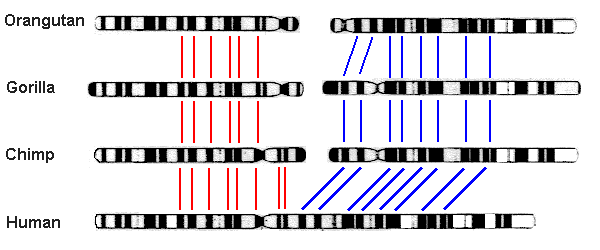An astonishing view into our similarities with chimps on a chromosomal level. (Image Source)

As a result, modeling how the evolution of chimps led to humans from the aspect of genomics is a monumental feat due to the number of different possibilities there could be for mutations. Mutations can consist of insertions (adding of base pairs), deletions (removing of base pairs), and alterations (changing of base pairs). When scaled to the billions of base pairs we have, the number becomes astronomical for the number of permutations that could’ve changed chimp DNA into human DNA.

Sequence alignment has solved this problem by allowing us to compare two genetic strands and find the sequence of mutations that led from one to the other. The goal of sequence alignment is to find homologous/similar sequences in the genome, and then base mutations off of the gaps that emerge between these sequences.

Because of the number of different evolutionary events that are possible, common sequence alignment algorithms attempt to minimize the number of events to explain the differences between two genetic sequences. Additionally, they use the heuristic that alterations are more common than insertions and deletions are.

## Terminology

• Sequence alignment: process of comparing two nucleotide strands to find the series of mutations that created one from the other
• Orthologous: event that leads to two organisms having a copy of the same gene (can use to trace back evolutionary roots of organisms)
• Paralogous: duplication event within a species (two members of the same species have similar genes)
• Global alignment: alignment of every element in the genetic strand
• Local alignment: alignment of regions that contain similar sequences
• NBAs (non-boring alignments): alignments where gaps are always paired with nucleotides (e.g. there isn’t a gap in both of the sequences)
• Gap penalty: penalty for creating a gap in one of the nucleotide sequences
• Substring: a continuous sequence of characters inside a string
• Subsequence: sequence that can be derived from a sequence by deleting some elements without changing the order of the remaining elements

# Considerations

## Minimizing Cost

In the following procedures, we need to minimize the “cost” as well as the number of events. As mentioned above, things that will increase cost are using an insertion (since alterations are more common than insertions) or substituting a purine for a pyrimidine (because purines are more likely to be switched with their corresponding purine).

Therefore, our goal is altered to find the least expensive, least operation sequence that transforms the initial sequence into the final sequence.

## Gap Penalty Models

In addition to accounting for mutations, there are extra considerations that must be made for gaps. For instance, biologically, the cost of creating a gap is much more expensive than that of extending an already created gap. There are a few different gap penalties currently used:

• Linear gap penalty: This uses a fixed cost for all gaps, regardless of the current gap length it is extending.
• Affine gap penalty: This imposes a large initial cost for opening a gap, but then a small incremental cost for each gap extension.
• General gap penalty: This uses a variety of different polynomial and linear functions to see which provides the best sequence alignment. Note this may drastically affect runtime.
• Frame-aware gap penalty: This tailors the cost function to account for disruptions in the coding frame; for instance, changes in a certain amino acid can cause phenotypic modifications.

Let’s express the gap penalty mathematically. If we let w(k) be the gap penalty for k gaps, we can see that the following emerges:

• Linear gap penalty: w(k) = p
• Affine gap penalty: w(k) = p + qk
• General gap penalty: w(k) = p + qk or w(k) = p + qk + rk²

The most simplistic method to find the number of mutations between two genetic sequences is to use the longest common substring between the two sequences. Then, you can put the substrings side-by-side in the two sequences, and explain the other nucleotides that are offset by insertions/alterations. However, this is clearly not the best solution, and it also runs very slowly, since you have to enumerate all possible alignments.

As seen in the example above, 6 mutations have been shown to be found in between S1 and S2, even though that is clearly not the most optimal solution and doesn’t minimize the number of steps between the two sequences.

# Longest Common Subsequence (Exponential)

One method to minimize the steps involves allowing gaps between subsequences and not just limiting it to substrings. Then, these substring parts can be aligned together, and mutations can be found in the gaps.

As seen in the example above, the mutations have been reduced from 6 mutations to 4, which means that our algorithm is getting better. However, the time complexity has greatly increased, from quadratic to exponential.

If considering NBAs (where gaps are always paired with nucleotides), and with sequences of length n and m where n > m, the number of alignments comes out to be (n+m) choose m, which is approximately 2^n alignments.

# Dynamic Programming

How can we reduce the runtime from exponential to quadratic once more while maintaining the same level of accuracy? The answer lies in dynamic programming, which allows us to utilize overlapping subproblems to solve a problem efficiently. For a dynamic programming problem, there are two major things to check for:

• Optimal Substructure: The optimal solution to a problem contains optimal solutions to subproblems of the problem.
• Overlapping Subproblems: Subproblems are repeated many times, so storing the answers to these subproblems will reduce computational complexity when running the model.

For dynamic programming, five major steps are needed:

• Find a parameterization of the problem, and determine the number of variables that are changing as the model is iterating.
• Ensure the subproblem space has polynomial time complexity and that there is extensive subprogram reusal.
• Determine an effective way to transverse through these variables.
• Determine a recursive formula. This sets up the substructure mentioned above where the optimal solution relies on optimal solutions to subproblems.
• Remember optimal solutions to subproblems, and make a polynomial representation of it.

## Dynamic Programming in Sequence Alignment

Dynamic programming can be used in sequence alignment by creating a matrix, where the column/row are the two sequences. The algorithm, in simple terms, systematically fills the table, finds optimal scores, and then traces back from the optimal score to find the optimal solution.

# Needleman-Wunsch Algorithm

## Setup

We now return back to the problem of the longest common subsequence between two sequences and how to reduce the time complexity from exponential to linear. Before doing, this a few variables need to be defined:

• S = {S1, S2, …, Sm} and T = {T1, T2, … Tn}: the two sequences
• d: the gap penalty cost (as explained above)
• s(x; y): the score of aligning a base x from S and a base y from T
• F: matrix where F (x, y) refers to the xth place in S and the yth place in T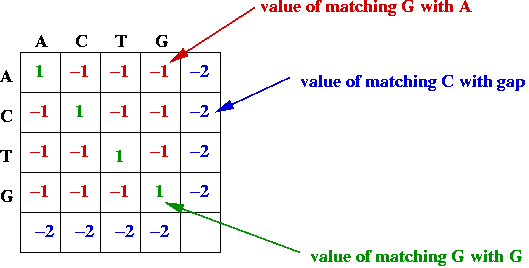How matrix F looks for S = ACTG and T = ACTG (Image Source)

This algorithm attempts to use a key part of dynamic programming: that you can keep track of the optimized sub-problems to solve the problem of finding the best subsequence. If there is an optimal alignment from F(0, 0) to F(m, n) (or the optimal alignment across both substrings), then this means that there is an optimal alignment between F(0, 0) to F(i, j) and F(i+1, j+1) and F(m, n) for any i in [0, m) and j in [0, n). This shows that every subpath in an optimal path has to also be optimal.

Additionally, it also tells us that a (m + 1) * (n + 1) space matrix is necessary, since F(i, j) stores the score for the optimal alignment at that position.

## Filling the Matrix

The first step is to initialize some rows and columns so that the algorithm can systematically fill data into other cells in the matrix. F(0, 0) is initialized with 0, because no alignments have been made yet. F(i, 0) = F(i-1, 0)-d, because a state change from F(i-1, 0) to F(i, 0) means that Sequence T had a gap, which contributes to a gap error penalty. Similarly, F(0, j) = F(0, j-1)-d, because a state change from F(0, j-1) to F(0, j) means that Sequence S had a gap.

The next step is to fill the matrix F with optimal scores. At each spot F(i, j), there are four possibilities that need to be considered:

• Sequence S has a gap at the current alignment: F(i, j-1)
• Sequence T has a gap at the current alignment: F(i-1, j)
• There is a nucleotide substitution at the current position: F (i-1, j-1)
• There is a match at the current position: F(i-1, j-1)

Each of these scenarios provide different scores. To maximize our final score and reduce our cost, we have to take the maximum from these scenarios.

The last step is our termination sequence, which ends at the bottom right or F(m, n). This is because this is the ending of both of the sequences, so there are no further changes to be made.

Note: It’s important when filling this matrix, that you remember how you got there (e.g. which cell gave you the maximum score). Therefore, along with storing the matrix F, pointers need to be stored showing which cells point to which.

## Traceback

After initializing the matrix, the final step is to traceback through the matrix starting at the bottom right until you get to the top left (or from F(m, n) to F(0, 0)). This will provide the optimal alignment, and determining what the path means will give you the longest common substring.

## Runtime

This algorithm takes O(mn) for both time and space complexity. This is because the instantiation of the matrix takes longer than the traceback, but since each instantiation of a cell takes O(1) time, for (m+1)*(n+1) cells, it takes O(mn) time. The same logic can be applied for space complexity.

Note: For non-linear gap complexities, the runtime could extend to O(mn(m+n)).

# Optimizations

## Bounded Dynamic Programming

Even with an O(mn) time complexity, that number could still be large, considering the billions of base pairs in the genome. Is there any way to make the model linear complexity?

One realization that can be made is only the squares that are close to the diagonals are being used for the traceback feature. This means that there is no need to instantiate the other numbers in the matrix. Granted, there may be some sequences that stray heavily from the diagonal, but this algorithm will likely capture relatively optimal alignments while ignoring alignments with too many gaps.

Mathematically, we can say that we limit the matrix to a distance W. This means that there will only be O((m+n) * W) time and space complexity, which means that there is now linear complexity. However, it’s important to remember that this will not guarantee optimal alignment anymore.

## Linear Space Alignment

Another observation that can be made is seeing that only the last updated column is needed to create the next column, which costs only O(m). However, even though the update is O(m), how can we make the traceback linear?

This can be done using divide and conquer, where a forward (starting at top left) and reverse (starting at bottom right) pass can be made that will converge in the middle column. The steps are as follows:

• Find the optimal score in the middle column by adding the two alignment scores for the columns next to it.
• Repeat the process by taking the two columns to the left and right of this.
• Use the previous middle column as a pointer to compute the maximum score for the middle column.

Because tracebacks require more computation, the total running time is O(mn) + O (1/2 mn) + O(1/4 mn) + … = O(2mn) = O(mn). However, this linear scalar of 2 pales in comparison to the polynomial reduction provided.

# Multiple Alignment

## Three-Sequence Alignment

Now that we’ve determined sequence alignment for two sequences, let’s see if we can extend the same logic to a series of three sequences S, T, and U. For these, there are seven different possibilities for each update of F:

• Three ways for two gaps in the alignment (3 choose 2 combinations)
• Three ways for one gap in the alignment (3 choose 1 combinations)
• One way for no gaps in the alignment (3 choose 0 combinations)

As such, the update rule is changed to the following:

Note that the time and space complexity expands accordingly to O(n^k) for k sequences of length n that need to be aligned. Consider using the various optimization algorithms discussed above to reduce this runtime.

## Progressive Multiple Alignment

Looking at three-sequence alignments, we can see that aligning multiple sequences will soon become unfeasible due to exponential growth. How can we reduce the growth of O(n^k) to something like O(k*n^2)?

First, let’s make an assumption that we know the evolutionary tree relating to each of our sequences and that our first two-sequence alignment is with the most closely-related sequences (this process is called seed alignment). Next, continue to align the next closest sequences to the seed, and each new alignment replaces the seed. This process continues until the final alignment is produced.

One thing to note is that this final alignment isn’t optimal, but produces a drastically shorter runtime than the n-sequence alignment algorithm that was mentioned above.

# Local Alignment

In this algorithm, we were considering how two sequences can have the longest common subsequence. This assumes that nucleotide groupings don’t switch places between mutations, and this can end up causing a huge number of mutations, when the actual result is much smaller.

As shown above, sequences of nucleotides in S and T can be switched around, even if there has been little to no mutations in between these two sequences. To code this up is relatively similar to the Needleman-Wunsch algorithm for global alignment. The initialization, iteration, and termination process are slightly altered:

• Initialization: Rather than saying F(i, 0) = F(i-1, 0)-d, F(i, 0) = 0 because note that local alignment states that there can be a switch in nucleotide sequences at any point in S or T. The applies to F(j, 0), which is also initialized to 0.
• Termination: Termination can now occur anywhere, as shown in the diagram above.
• Iteration: Because termination can now occur anywhere, we want to penalize negative scores heavily. Therefore, we now add a new constraint, where if the maximum is negative, then we make F(i, j) = 0.

# Semi-Global Alignment

Semi-global looks at an alignment of a substring s of sequence S with a substring of t of sequence T.

Similar to local alignment, there’s slight changes with the code from the Needleman-Wunsch algorithm that allow for this semi-global alignment:

• Initialization: Same as local alignment
• Termination: Termination can happen on anything in the bottom row or the right column. This is because these are the places where one of the sequences, S or T, stops. Since we are looking for semi-global alignment, once the sequence stops, there’s no way to go further, similar to global alignment.

# TL;DR

• Sequence alignment allows us to compare two genetic strands and determine what mutations led one to another.
• During sequence alignments, certain costs have to be placed on gap sizes and different nucleotide alterations so that the sequence alignment makes more sense probabilistically and biologically.
• The most simplistic sequence alignment algorithm, the most common substring, has the least accuracy but one of the fastest runtimes (quadratic). The most common subsequence has a higher accuracy but an extremely slow runtime (exponential).
• Dynamic programming allows us to use repeating optimal subsequences to reduce the computation runtime of the model. The Needleman-Wunsch algorithm creates a matrix of scores for each subalignment, and finds the optimal alignment by tracing back through the maximum scores. The time and space complexity for this algorithm is quadratic.
• Optimizations, such as bounded space programing and linear space alignment, exist to make the runtime linear.
• The Needleman-Wunsch algorithm can be extended to sequence alignment for multiple sequences.
• Instead of matching whole sequences together, certain sections of the sequences can be matched together to increase accuracy of the model and provide a better view of the evolutionary pathway between the two sequences provided.

If you want to talk more, schedule a meeting: Calendly! For information about projects that I am currently working on, consider subscribing to my newsletter! Here’s the link to subscribe. If you’re interested in connecting, follow me on Linkedin, Github, and Medium.

--

--

--

## More from Analytics Vidhya

Analytics Vidhya is a community of Analytics and Data Science professionals. We are building the next-gen data science ecosystem https://www.analyticsvidhya.com

## Did Researchers Bring Dead Pigs Back to Life? And Would That Be OK?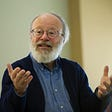## Nitrates can stimulate cancer, mainly related to the digestive system.## Five Stages of Roasting 2## [Throwback] APBioNET Newsletter 2019 (Issue 4)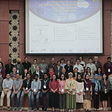## 6 Crazy Weather Modification Projects that are Real## The Gendered Brain: A Murky Debate## The future belongs to the curious and our future is in the minds of our young.## Top 10 Innovations 2019, Q1## Painless Simulation of Clinical Trial Data for Linear Regression in R## Normalization — The Key To Scoring Cybersecurity Health Of Organizations Of Varying Size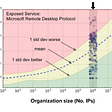## A Catalogue of Genomic Data Diversity Initiatives